# Class 10 Science|physics Conceptual's Questions for Electricity

Given below are the Class 10 Science Conceptual Questions for electricity
(a) Conceptual Short questions
(b) Multiple Choice Questions
(b) Fill in the blank's
(c) Crossword
(d) True and False

## Conceptual Short questions

Question 1
Give us the factors on which resistance of the conductors depends
Solution
The resistance of a conductor depends
(a) Length
(b) Area of its cross-section
(c) Nature of the material

Question 2
Write down the difference between Ammeters and Voltmeters?
Solution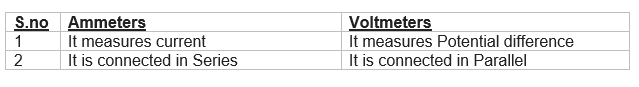Question 3
Why is Tungsten used almost exclusively for filament of electric lamps?
Solution
Melting point of tungsten is very high so it is used for making filament of electric lamps

Question 4
What happens to resistance of the conductor?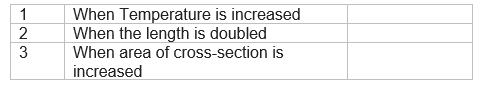Solution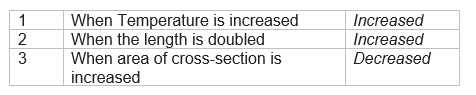Question 5
What is the difference between Open circuits and closed circuits?
Solution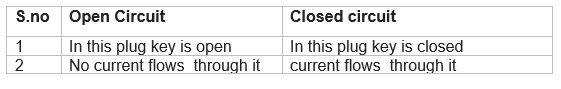Question 6
Why does the cord of an electric heater not glow while heating element does?
Solution
The cord of electric Heater is made of cooper which has extremely low resistance due to this negligible resistance heat is produced in it
The heating element of an electric heater is made of nichrome wire; It glows because large amount of heat is produced due to its high resistance

Question 7
Why do we use copper wire as connecting wires in the circuit?
Solution
This is because Copper has very low resistivity

Question 8
What does an electric circuit mean?
Solution
Combination of electrical devices connected by conducting wires is called an electric circuit.

Question 9
Define the unit of electric current.
Solution
The rate of flow of charge is called electric current and its S. I. unit is ampere. One ampere current is defined as below:
"If one coulomb charge flows through a conductor per second the strength of current is called one ampere."

Question 10
Name a device that helps to maintain a potential difference across a conductor.
Solution
Cell is the device that helps to maintain a potential difference, across a conductor.

Question 11
What is meant by saying that the potential difference between two points is 1 V?
Solution
Potential difference of 1 volt means that the rate of doing work during transfer of per coulomb charge is joule.

Question 12
Will current flow more easily a thick wire or a thin wire of the same material, when connected to the same source? Why?
Solution
The current will flow easily through the thick the wire because the resistance of thick wire is less than thin wire.
$R \propto \frac{1}{A}$

Question 13
Let the resistance of an electrical component remains constant while the potential difference across the two ends of the componenets decreases to half of its former value. What change will occur in the current through it?
Solution
At constant temperature and resistance:
$V \propto I$

Question 14
Why are coils of electric toasters and electric irons, made of an alloy rather than a pure metal?
Solution
Alloys have more resistance then constituent metals. So, they are heated up easily due to the heating effect of electric current.

Question 15
(a)Which among iron and mercury is a better conductor?
(b) Which material is the best conductor?
Solution
(a) iron
(b) Silver.

Question 16
What are the advantages of connecting electrical devices in parallel with the battery instead of connecting them in series?
Solution
The equivalent resistance of resistances connected in parallel is less than even the smallest resistance connected in parallel, so large current is obtained in the ciruit for the same potential difference. Since, the equivalent resistance is less, therefore, loss of energy due to resistance of conductor is less. These are the advantages of connecting the resistances in parallel.

Question 17
Why does the cord of an electric heater not glow while the heating element does ?
Solution
We know, $H = I^2 Rt$
$H \propto\ R$
The resistance of heating elements very high, so more electrical energy is converted into heat energy, hence it glows. But the cord has very low resistance, so it does not glow.

Question 18
What determines the rate at which energy is delivered by a current?
Solution
Electrical power determines the rate at which energy is delivered by a current.

## Multiple Choice Questions

Question 19
Which of the following represents voltage?
(a) $\frac {\text {Work done}}{\text {Current} \times \text {Time}}$
(b) $\text{Work done} \times \text{Charge}$
(c) $\frac {\text {Work done} \times \text{Time}}{\text {Current}}$
(d) $\text{Work done} \times \text{Charge} \times \text{Time}$
Solution
(a)

Question 20
Two resistors of resistance 1 ohm and 2 ohm when connected to a battery will have
(a) same current flowing through them when connected in parallel
(b) same current flowing through them when connected in series
(c) same potential difference across them when connected in series
(d) different potential difference across them when connected in parallel
Solution
(b)

Question 21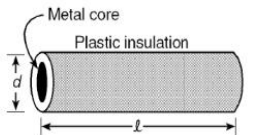Plastic insulation surrounds a wire having diameter d and length l as shown above. A decrease in the resistance of the wire would be produced by an increase in the
(a) length l of the wire
(b) diameter d of the wire
(c) temperature of the wire
(d) thickness of the plastic insulation
Solution
(b)

Question 22
A complete circuit is left on for several minutes, causing the connecting copper wire to become hot. As the temperature of the wire increases, the electrical resistance of the wire
(a) decreases.
(b) remains the same.
(c) increases.
(d) increases for some time and then decreases .
Solution
(c)

Question 23
Two LED bulbs of 12W and 6W are connected in series. If the current through 12W bulb is 0.06A the current through 6W bulb will be :
(a) 0.04A
(b) 0.06A
(c) 0.08A
(d) 0.12A
Solution
(b)

Question 24
The resistance of a resistor is reduced to half of its initial value. If other parameters of the electrical circuit remain unaltered, the amount of heat produced in the resistor will become :
(a) four times
(b) one fourth
(c) half
(d) two times
Solution
(d)

## Fill in the blanks

Question 25
1. The positively charged electrode in an electrolytic cell is called ________.
2. The positively electrode in an electrochemical cell is known as ___________.
3. The potential difference between the ends of the wire of resistance 1 ohm is doubled, the electric power across the wire will ______ by _____.
4. The commercial unit of electric power is _________.
5. Mercury becomes super conductor when temperature is dropped to _______.
6. During the electrolysis of a salt solution,the electricity is carried by _______ and________.

## Crossword Puzzle

Question 26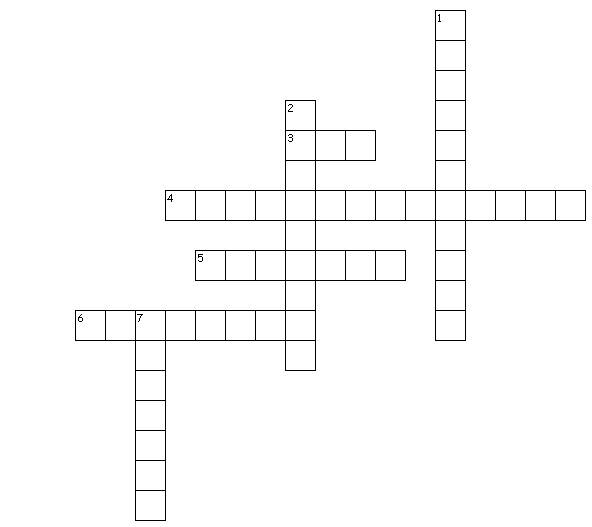Across
3. This law states that current is directly proportional to voltage applied
4. This is a process for depositing a thin layer of desired metal over another metal
5. Instrument used to measure current
6. This alloy is used for making heating elements of electrical appliances

Down
1. Substance having infinite resistance
2. Instrument used to measure Voltage
7. This is the unit of charge

## True and False

Question 27
True and False Statements
(a) Series arrangement is used in domestic circuits
(b) Resistance of the wire is directly proportional to the length of the wire
(c) The graph between V and I is a straight line
(d) A thick wire of length L will have less resistance than the thin wire of same length and same material
Solution
(a) False, Parallel arrangement are used in domestic circuits
(b) True
(c) True
(d) True